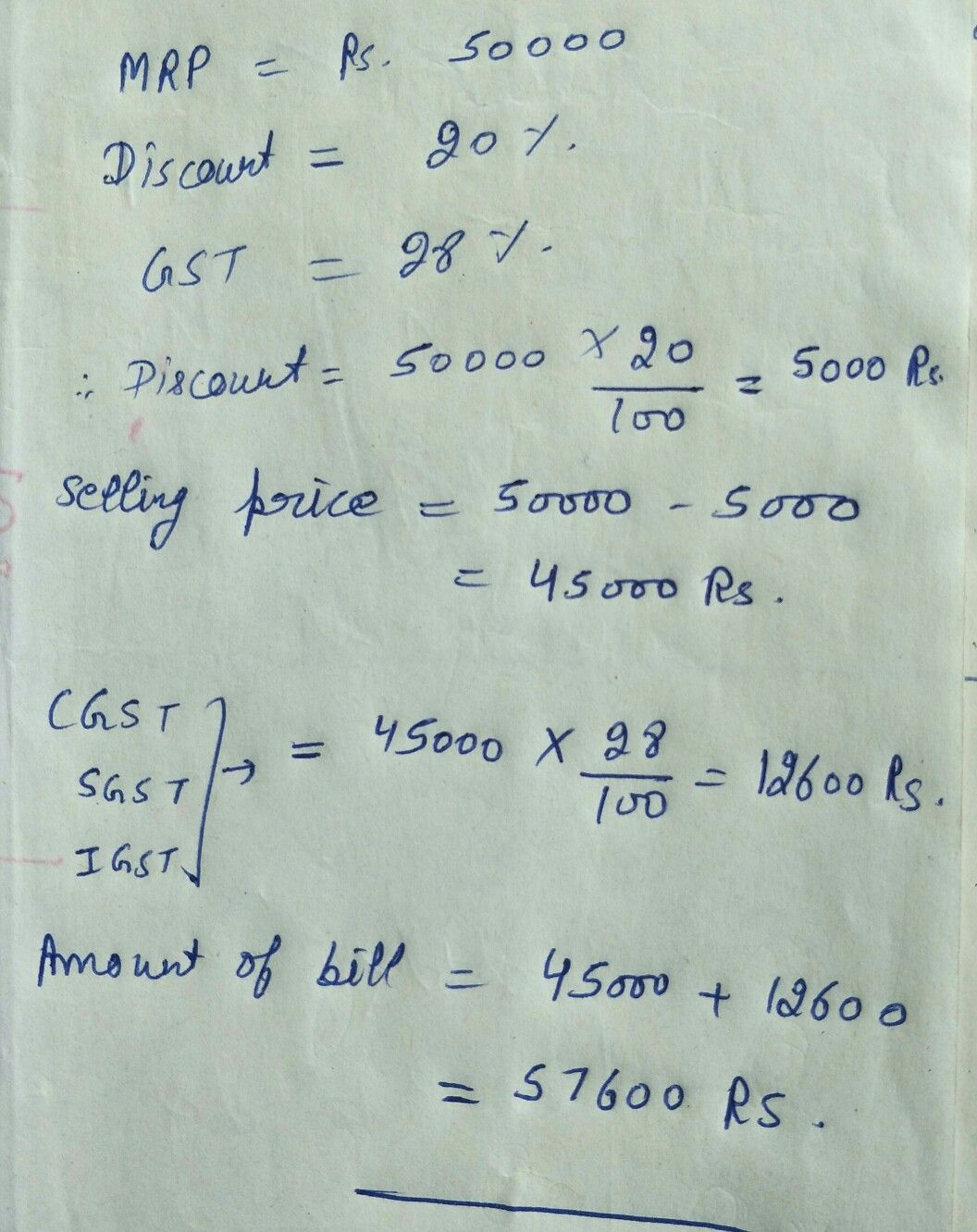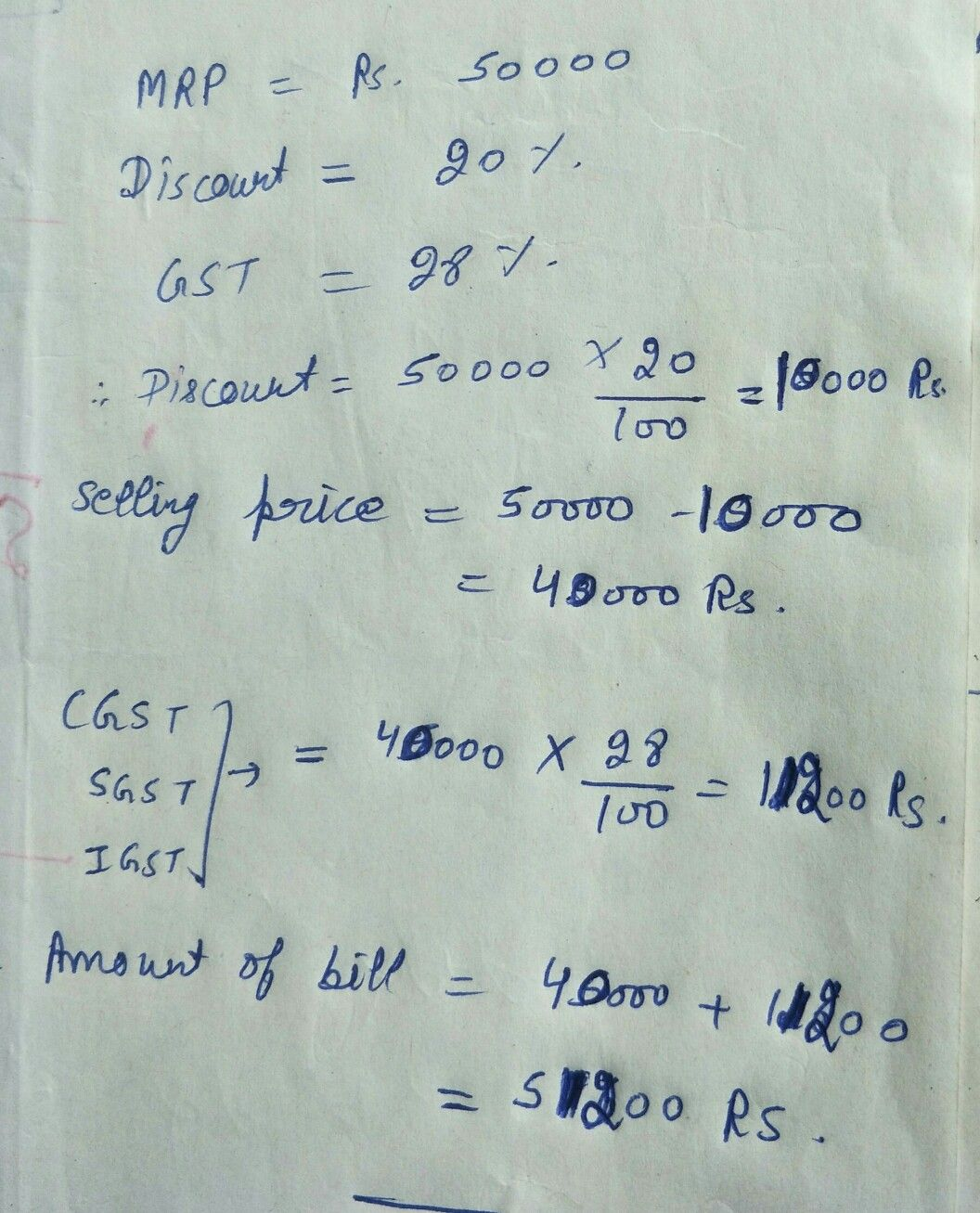Symbol
Problem$1s1$ $1\left(A1$ $2$ For the following transaction from Delhi to Jaipur, fill in the blanks to find the amount of bill $MRP=z$ $50.000$ Discount $=20%$ $GsT=2896$ Discount $=$ Selling price $=$ (discounted value) $C51$ $=$ $sG5T$ $=$ $1GsT=$ Amount of bill $=$ $z$
10th-13th grade
Other
Search count: 106
SolutionQanda teacher - AnoopDhillStudent
sir u calculated discount wrong but no worriesQanda teacher - AnoopDhill
Yes discount calculations is 10000. Calculations mistakeStudent
thank you! But next time provide more explanation including neat handwriting with good visibility of solution .We appreciate your work! keep maintaining good quality of results:)